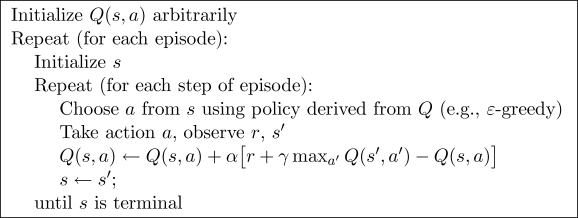# 小例子

## 要点 ¶

-o---T
# T 就是宝藏的位置, o 是探索者的位置


Q-learning 是一种记录行为值 (Q value) 的方法, 每种在一定状态的行为都会有一个值 Q(s, a), 就是说 行为 as 状态的值是 Q(s, a). s 在上面的探索者游戏中, 就是 o 所在的地点了. 而每一个地点探索者都能做出两个行为 left/right, 这就是探索者的所有可行的 a 啦.

## 预设值 ¶

import numpy as np
import pandas as pd
import time

N_STATES = 6   # 1维世界的宽度
ACTIONS = ['left', 'right']     # 探索者的可用动作
EPSILON = 0.9   # 贪婪度 greedy
ALPHA = 0.1     # 学习率
GAMMA = 0.9    # 奖励递减值
MAX_EPISODES = 13   # 最大回合数
FRESH_TIME = 0.3    # 移动间隔时间


## Q 表 ¶

def build_q_table(n_states, actions):
table = pd.DataFrame(
np.zeros((n_states, len(actions))),     # q_table 全 0 初始
columns=actions,    # columns 对应的是行为名称
)
return table

# q_table:
"""
left  right
0   0.0    0.0
1   0.0    0.0
2   0.0    0.0
3   0.0    0.0
4   0.0    0.0
5   0.0    0.0
"""


## 定义动作 ¶

# 在某个 state 地点, 选择行为
def choose_action(state, q_table):
state_actions = q_table.iloc[state, :]  # 选出这个 state 的所有 action 值
if (np.random.uniform() > EPSILON) or (state_actions.all() == 0):  # 非贪婪 or 或者这个 state 还没有探索过
action_name = np.random.choice(ACTIONS)
else:
action_name = state_actions.argmax()    # 贪婪模式
return action_name


## 环境反馈 S_, R ¶

def get_env_feedback(S, A):
# This is how agent will interact with the environment
if A == 'right':    # move right
if S == N_STATES - 2:   # terminate
S_ = 'terminal'
R = 1
else:
S_ = S + 1
R = 0
else:   # move left
R = 0
if S == 0:
S_ = S  # reach the wall
else:
S_ = S - 1
return S_, R


## 环境更新 ¶

def update_env(S, episode, step_counter):
# This is how environment be updated
env_list = ['-']*(N_STATES-1) + ['T']   # '---------T' our environment
if S == 'terminal':
interaction = 'Episode %s: total_steps = %s' % (episode+1, step_counter)
print('\r{}'.format(interaction), end='')
time.sleep(2)
print('\r                                ', end='')
else:
env_list[S] = 'o'
interaction = ''.join(env_list)
print('\r{}'.format(interaction), end='')
time.sleep(FRESH_TIME)


## 强化学习主循环 ¶def rl():
q_table = build_q_table(N_STATES, ACTIONS)  # 初始 q table
for episode in range(MAX_EPISODES):     # 回合
step_counter = 0
S = 0   # 回合初始位置
is_terminated = False   # 是否回合结束
update_env(S, episode, step_counter)    # 环境更新
while not is_terminated:

A = choose_action(S, q_table)   # 选行为
S_, R = get_env_feedback(S, A)  # 实施行为并得到环境的反馈
q_predict = q_table.loc[S, A]    # 估算的(状态-行为)值
if S_ != 'terminal':
q_target = R + GAMMA * q_table.iloc[S_, :].max()   #  实际的(状态-行为)值 (回合没结束)
else:
q_target = R     #  实际的(状态-行为)值 (回合结束)
is_terminated = True    # terminate this episode

q_table.loc[S, A] += ALPHA * (q_target - q_predict)  #  q_table 更新
S = S_  # 探索者移动到下一个 state

update_env(S, episode, step_counter+1)  # 环境更新

step_counter += 1
return q_table


if __name__ == "__main__":
q_table = rl()
print('\r\nQ-table:\n')
print(q_table)